# Physics 751 Homework #9

1.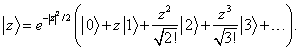Exercise: Check that this state is correctly normalized, and is an eigenstate of.

2.  Prove using an algebraic identity thatis an eigenstate of.  Is it also an eigenstate of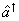?  Prove your assertion.

2.  Prove that if,

the unit operator3.  Prove that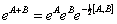is correct up to terms A3 and B3 by expanding the exponentials on both sides and comparing.

4.  How does a (position) translation operator affect a wave function expressed in momentum space,?   What is the operator that shifts the momentum space wave functionto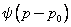?  How does that operator change?

5.  Prove:by writing the Taylor series forand finding the successive derivatives at the origin.

A unitary squeeze operator is defined by:Use the result forabove to prove that: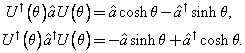Deduce that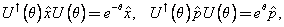so for positive θ, the wave function is scaled down—squeezed—in x space, but simultaneously expanded in p space, as it must be, since it was a minimum uncertainty packet.

Is it still a minimum uncertainty packet?   Is it still an eigenstate of the annihilation operator?   If not, what is it an eigenstate of?  How do you think it develops in time?